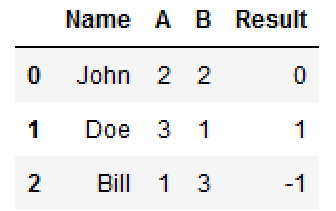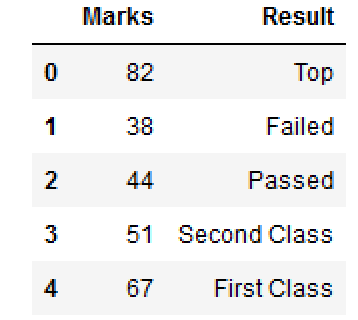# New dataframe column based on a given condition

There are times when you would like to add a new DataFrame column based on some condition . Actually, there does not exist any Pandas library function to achieve this method directly.

Suppose you have a DataFrame like this:

Name A B 0 John 2 2 1 Doe 3 1 2 Bill 1 3
You want to create a new column "Result" based on the following condition:
1. A == B: 0
2. A > B: 1
3. A < B: -1

So, by applying above condition, DataFrame should be:

Name A B Result 0 John 2 2 0 1 Doe 3 1 1 2 Bill 1 3 -1
How yo can achieve above condition through Pandas DataFrame operation?

Lets create a DataFrame..

import pandas as pd import numpy as np df = pd.DataFrame() df['Name'] = ['John', 'Doe', 'Bill'] df['A'] = [2,3,1] df['B'] = [2, 1, 3] df
Name A B 0 John 2 2 1 Doe 3 1 2 Bill 1 3

## Vectorized Version

df['Result'] = np.where( df['A'] == df['B'], 0, np.where( df['A'] > df['B'], 1, -1))
Full Source
import pandas as pd import numpy as np df = pd.DataFrame() df['Name'] = ['John', 'Doe', 'Bill'] df['A'] = [2,3,1] df['B'] = [2, 1, 3] df df['Result'] = np.where( df['A'] == df['B'], 0, np.where( df['A'] > df['B'], 1, -1)) dfName A B Result 0 John 2 2 0 1 Doe 3 1 1 2 Bill 1 3 -1

## Using if..else

def f(row): if row['A'] == row['B']: val = 0 elif row['A'] > row['B']: val = 1 else: val = -1 return val df['Result'] = df.apply(f, axis=1)
Full Source
import pandas as pd import numpy as np df = pd.DataFrame() df['Name'] = ['John', 'Doe', 'Bill'] df['A'] = [2,3,1] df['B'] = [2, 1, 3] def f(row): if row['A'] == row['B']: val = 0 elif row['A'] > row['B']: val = 1 else: val = -1 return val df['Result'] = df.apply(f, axis=1) df
Name A B Result 0 John 2 2 0 1 Doe 3 1 1 2 Bill 1 3 -1

## Operation on Single column

Suppose, you have a DataFrame like this:

Marks 0 82 1 38 2 44 3 51 4 67

You would like to add one more column for Result based on certain conditions.

1. Marks <= 30 : Failed
2. Marks >= 40 and <=49 : Passed
3. Marks >= 50 and <=59 : Second Class
4. Marks >= 60 and <=79 : First Class
5. Marks >= 80 and <=100 : Top
How you can create a dataFrame column based on the above condition using DataFrame.loc[] .
df.loc[df['Marks'] <= 39, 'Result'] = 'Failed' df.loc[(df['Marks'] >= 40) & (df['Marks'] <= 49) , 'Result'] = 'Passed' df.loc[(df['Marks'] >= 50) & (df['Marks'] <= 59) , 'Result'] = 'Second Class' df.loc[(df['Marks'] >= 60) & (df['Marks'] <= 79) , 'Result'] = 'First Class' df.loc[(df['Marks'] >= 80) & (df['Marks'] <= 100) , 'Result'] = 'Top'
Full Source
numbers = {'Marks': [82,38,44,51,67]} df = pd.DataFrame(numbers,columns=['Marks']) df.loc[df['Marks'] <= 39, 'Result'] = 'Failed' df.loc[(df['Marks'] >= 40) & (df['Marks'] <= 49) , 'Result'] = 'Passed' df.loc[(df['Marks'] >= 50) & (df['Marks'] <= 59) , 'Result'] = 'Second Class' df.loc[(df['Marks'] >= 60) & (df['Marks'] <= 79) , 'Result'] = 'First Class' df.loc[(df['Marks'] >= 80) & (df['Marks'] <= 100) , 'Result'] = 'Top' print (df)Marks Result 0 82 Top 1 38 Failed 2 44 Passed 3 51 Second Class 4 67 First Class